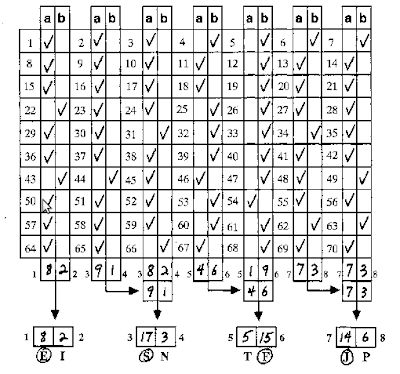## Saturday, April 9, 2011

### Myers-Briggs Test in Javascript / Python

Hunch.com apparently uses this method - Myers-Briggs Test is a psychology, profile evaluation system, expanded upon later by David Keirsey. We coded the evaluation scheme in Python, making a few additions. In the original version in David Keirsey book Please Understand Me II, the answer to each question is either A, or B, these choices in Python code as -1, +1 then sum up appropiate array values. Recent versions of this questionaire carry more (sometimes even five) choices. We noticed an additional 'neutral' choice can made an improvement, our version carries 3 answers. Eval code substitutes -1 for A, +1 for B, and 0 for neutral.An example of the evaluation algorithm Keirsey uses in his book is above. We simply generate indexes that correspond to the columns seen above (answers arrive in a straight list, numbered from 1 to 70), then do the addition.
```def calculate_mb(choices):

new_choices = []

for i in range(1,8):
new_choices.append([int(choices[j-1]) for j in range(i,71,7) ])

res = list("XXXX")

ei = sum(new_choices)
if ei < 0: res = 'E'
else: res = 'I'

sn = sum(new_choices) + sum(new_choices)
if sn < 0: res = 'S'
else: res = 'N'

tf = sum(new_choices) + sum(new_choices)
if tf < 0: res = 'T'
else: res = 'F'

jp = sum(new_choices) + sum(new_choices)
if jp < 0: res = 'J'
else: res = 'P'

logging.debug(choices)

return str(''.join(res))```

Another version, in HTML using Javascript, can be found here.